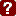Help

6-Couple Sequence Indicator - Rectangle

For symmetric 6-couple rectangular choreography, there are 64 possible sequence states.

The state is indicated as two numbers separated by a dash (e.g., B-G), where B indicates the boy's state, and G indicates the girl's state.

Values for B and G:

```	 0  -->  in sequence.

14  -->  1 and 4 swapped.
25  -->  2 and 5 swapped.
36  -->  3 and 6 swapped.

12  -->  1 and 2 swapped.  (also 4 and 5).
13  -->  1 and 3 swapped.  (also 4 and 6).
23  -->  2 and 3 swapped.  (also 5 and 6).
34  -->  3 and 4 swapped.  (also 6 and 1).

88  -->  asymmetric.
99  -->  unable to determine sequence.```